How Cheenta works to ensure student success?
Explore the Back-Story

# Test of Mathematics Solution Subjective 76 - Range of a Rational PolynomialThis is a Test of Mathematics Solution Subjective 76 (from ISI Entrance). The book, Test of Mathematics at 10+2 Level is Published by East West Press. This problem book is indispensable for the preparation of I.S.I. B.Stat and B.Math Entrance.

## Problem

Find the set of all values of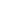such that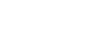can take all real values.

## Solution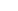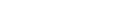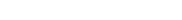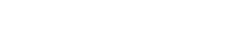Now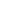takes all real values if discriminant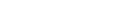is allways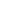.
So now we have to find the all values ofsuch thatfor all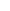|R.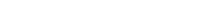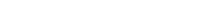...(i)
Now this is a equation of upside open parabola. If the discriminant is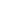of equation (i) thenwill always positive.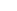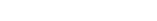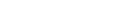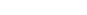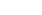Conclusion:

If, thencan take all the values as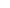varies over |R.This is a Test of Mathematics Solution Subjective 76 (from ISI Entrance). The book, Test of Mathematics at 10+2 Level is Published by East West Press. This problem book is indispensable for the preparation of I.S.I. B.Stat and B.Math Entrance.

## Problem

Find the set of all values ofsuch thatcan take all real values.

## SolutionNowtakes all real values if discriminantis allways.
So now we have to find the all values ofsuch thatfor all|R....(i)
Now this is a equation of upside open parabola. If the discriminant isof equation (i) thenwill always positive.Conclusion:

If, thencan take all the values asvaries over |R.

### Knowledge Partner